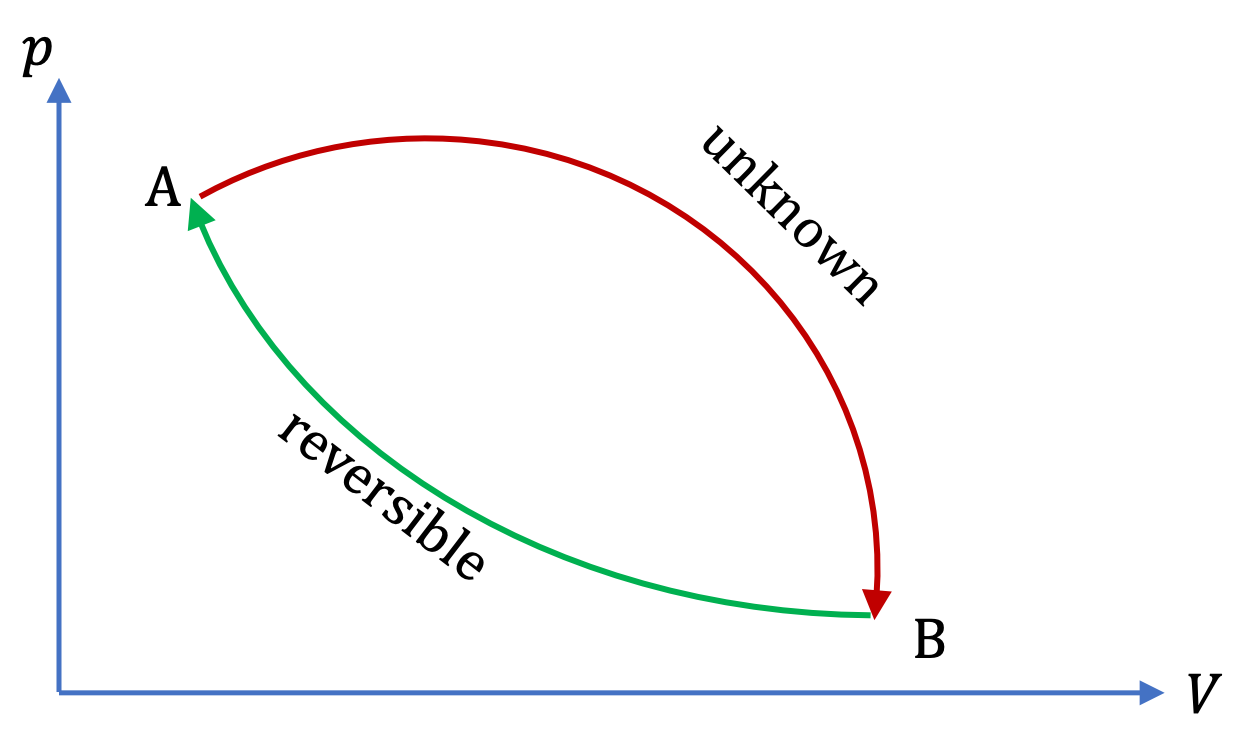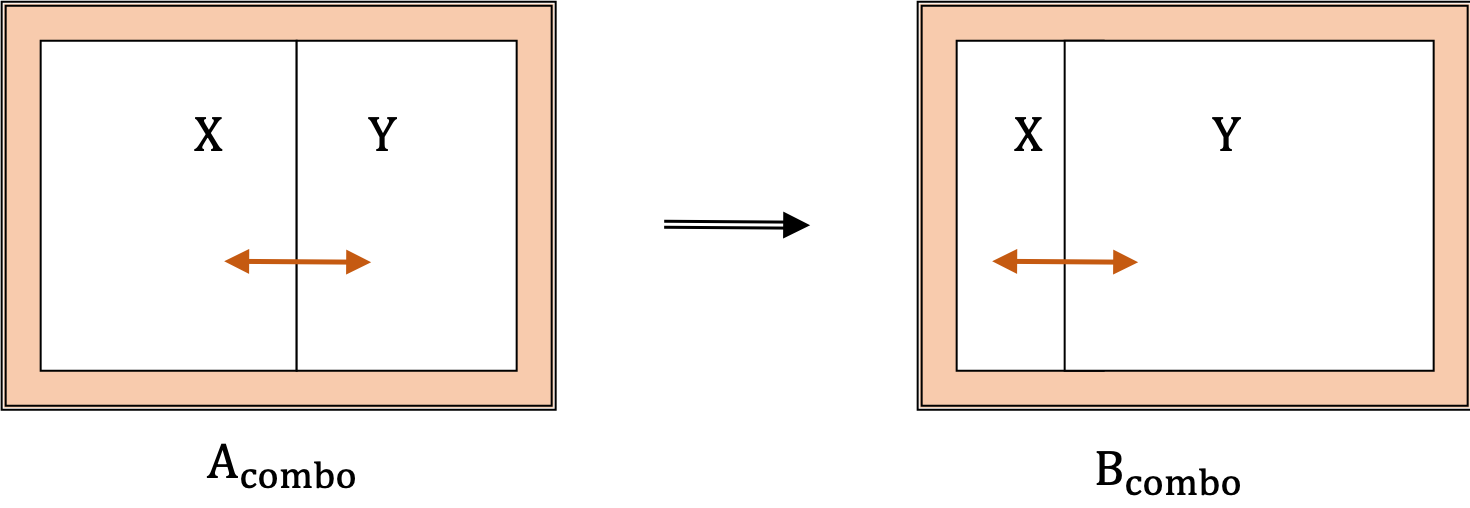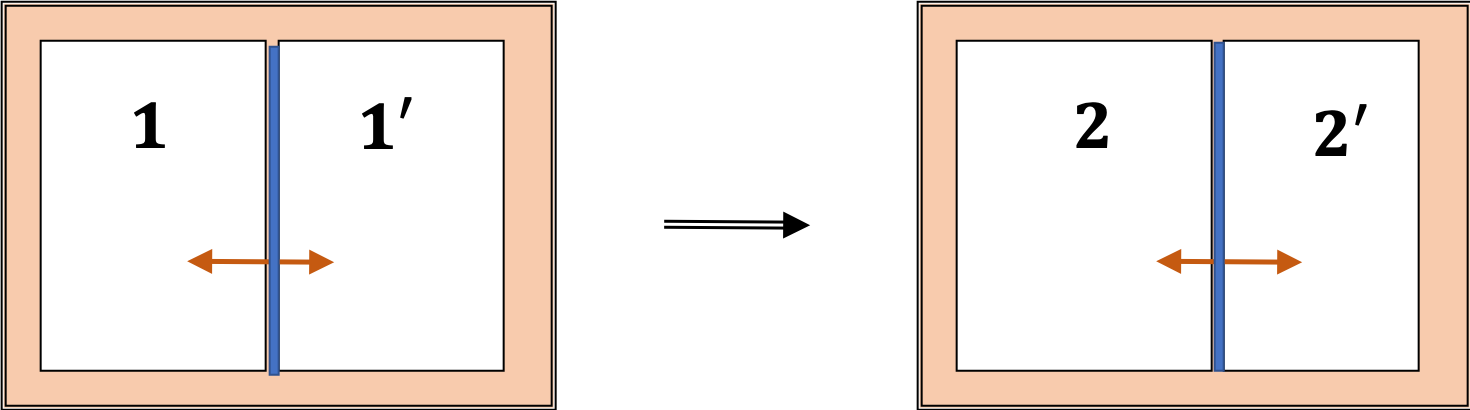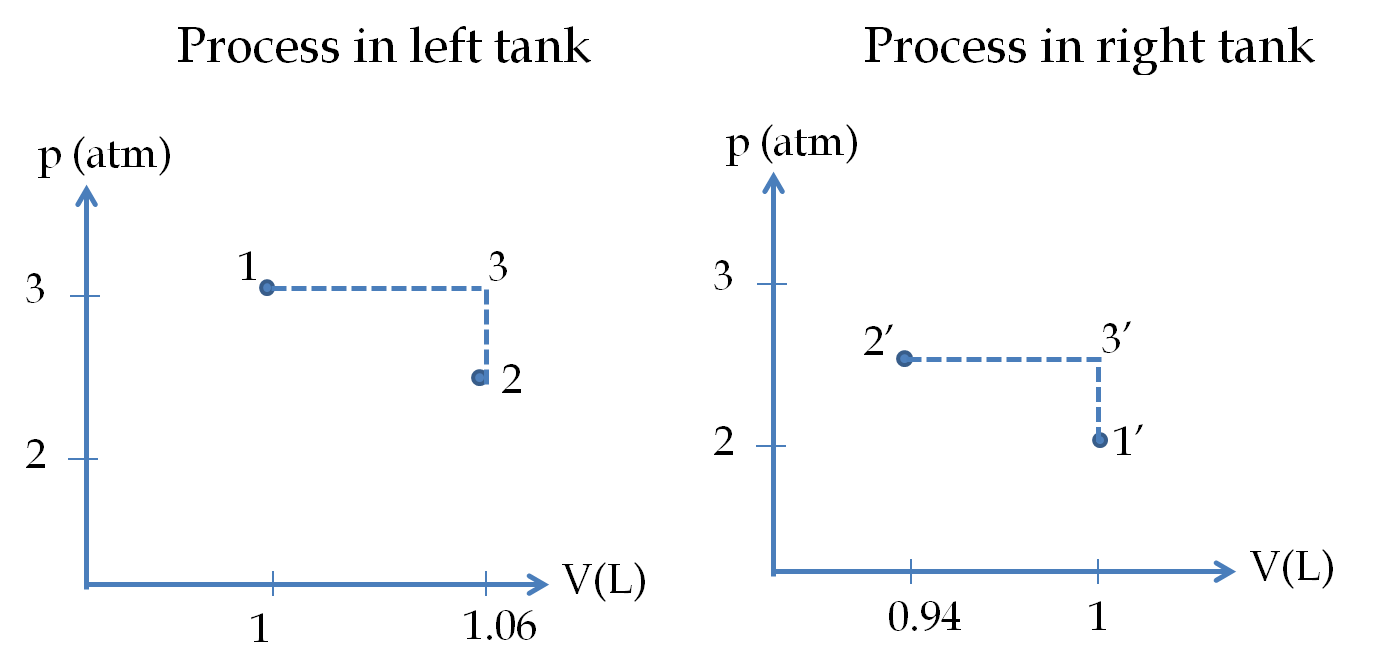## Section26.5The Second Law and Entropy

We show below that entropy change for an isolated system is always positive for all real processes. In the case of interacting system, then we can combine system with environment to get an isolated system. This will give the entropy change of system more then compensated by opposite change in entropy of the environment.

\begin{equation*} \Delta S_\text{system} + \Delta S_\text{environment} \ge 0. \end{equation*}

### Subsection26.5.1(Calculus) Entropy Change of Universe

Clausius proved that, for any cyclic process, whether it be reversible or irreversible, the following inequality must hold true.

\begin{equation} \oint \dfrac{dQ}{T}\le 0\ \ \ \textrm{(All cyclic processes)}\tag{26.5.1} \end{equation}

Here, the equality holds only when the process is reversible.

Now, let us consider a cyclic process that is made up of an unknown process from state A to state B, and a reversible process from B to A as shown in the figure.When Clausius's result is applied to this cyclic process it yields the following result.

\begin{equation} \left( \int_A^B \dfrac{dQ}{T} \right)_\text{unknown} + \left( \int_B^A \dfrac{dQ}{T} \right)_\text{rev} \le 0,\tag{26.5.2} \end{equation}

where the equality will be the case if both processes were reversible. Now, from the definition of entropy, the integral over the reversible process is equal to the difference in entropy of states A and B.

\begin{equation} \left( \int_A^B \frac{dQ}{T} \right)_{\text{unknown}} + S(A) - S(B) \le 0.\tag{26.5.3} \end{equation}

Moving the entropy change on the other side of the inequality, we obtain the following for change of entropy for the A to B process.

\begin{equation} S(B) - S(A) \ge \left( \int_A^B \frac{dQ}{T} \right)_{\text{unknown}}. \label{eq-second-law-entropy}\tag{26.5.4} \end{equation}

Now, we apply this result to a combined system consisting of two subsystems, X and Y, which can interact thermally as shown in Figure 26.5.1, but they are thermally insulated from the rest of the universe. Consider a state change process in which the combined system changes from state A$_\text{combo}$ to state B$_\text{combo}\text{.}$Figure 26.5.1. An isolated combined system undergoing change from a state A$_\text{combo}$ to another state B$_\text{combo}\text{.}$

Since there is no heat exchange with the outside world, the right side of Eq. (26.5.4) is zero. The final entropy of the combined system $S(B)$ will then be greater than the entropy of the initial state $S(A)\text{.}$

\begin{equation} S(B) - S(A) \ge 0\ \ \text{(Isolated system, state A to B transformation)}, \label{eq-second-law-entropy-2}\tag{26.5.5} \end{equation}

where the equality sign holds when A$_\text{combo}$ to B$_\text{combo}$ transformation occurs by a reversible process. This means that if the subsystem X is our finite system and Y the rest of the universe, then the net entropy of the universe does not decrease in any process.

\begin{equation} \Delta S_\text{system} + \Delta S_\text{environment} \ge 0.\label{eq-second-law-entropy-3}\tag{26.5.6} \end{equation}

If the process is reversible, then the reduction in entropy of the system will be balanced by increase in entropy of the environment and vice-versa. If the process is irreversible, however, then the increase in entropy of the environment will be more than any reduction in the entropy of the system, or vice-versa. This leads to the following statement of second law of thermodynamics in terms of entropy.

The entropy of the universe cannot decrease.

A nonconducting cylinder of total volume 2 L closed at both ends is divided into two parts by a diathermal frictionless circular wall. Initially the piston is clamped in the middle with the Nitrogen gas, assumed to be an ideal gas of $C_V=\dfrac{5}{2}R\text{,}$ at different temperatures and pressures on the two side. The initial pressure and temperatures on the left and right sides are (3 atm, 330 K) and (2 atm, 250 K) respectively.

(a) Find the temperature and pressure of the final state when the two sides are in thermal and mechanical equilibrium.

(b) Find the net change in the entropy of the gas.Hint

(a) at mechanical equilibrium pressure on the two sides must become equal. (b) replace the actual process between the initial and final states by constant pressure and constant volume processes since computation of entropy on them will be easier to compute.

(a) $2.51\ \textrm{atm}\text{,}$ $V_1 = 1.064\ \textrm{L}\text{,}$ $V_2 = 0.936\ \textrm{L}.$ (b) see the solution.

Solution 1 (a)

(a) From the given data on the left and right chambers in the ideal gas law for each we obtain

\begin{align*} \amp n_1 = \frac{p_1 (V_0/2)}{RT_1} = 0.111\ \textrm{mol}.\\ \amp n_1 = \frac{p_2 (V_0/2)}{RT_2} = 0.0976\ \textrm{mol}. \end{align*}

Now, we find the common pressure $p$ and temperature $T$ values in the two chambers in the final state. To find the temperature we note that total energy of the two systems will not change since although the gas on the sides interact with each other, the two together are isolated from other systems.

\begin{equation*} \Delta U = \Delta U_1 + \Delta U_2 = 0. \end{equation*}

The change in the internal energies of the two sides separately will be

\begin{align*} \amp \Delta U_1 = n_1 C_V (T - T_1).\\ \amp \Delta U_2 = n_2 C_V (T - T_2). \end{align*}

Therefore,

\begin{equation*} T = \frac{ n_1 T_1 + n_2 T_2}{ n_1 + n_2 } = \frac{ 0.111 \times 330+ 0.0976\times 250 }{ 0.111 + 0.0976 } = 293\ \textrm{K}. \end{equation*}

Let $V_1$ and $V_2$ be the volumes on the left and right sides at equilibrium. Let us denote the total volume by $V_0\text{.}$ Then, we have

\begin{equation} V_1 + V_2 = V_0. \label{eq-ch20-pr-8-1}\tag{26.5.7} \end{equation}

The volumes on the two sides are also given by the ideal gas laws on the two sides.

\begin{align*} \amp V_1 = n_1 \frac{R T}{p} \equiv n_1 x.\\ \amp V_2 = n_2 \frac{R T}{p} = n_2 x. \end{align*}

Put these in Eq. (26.5.7) to obtain

\begin{equation*} V_1 = 1.064\ \textrm{L},\ \ V_2 = 0.936\ \textrm{L}. \end{equation*}

Now, applying the ideal gas law on either of the chamber in the final state gives

\begin{equation*} p = \frac{n_1RT}{V_1} = \frac{0.111\ \textrm{mol} \times 0.082\ \textrm{L.atm/mol.K}\times 293}{1.064\ \textrm{L}} = 2.51\ \textrm{atm}. \end{equation*}
Solution 2 (b)

(b) To compute the entropy change, we envision reversible steps that lead to the same change in state but where the computation of entropy changes are easier to compute. The following figure gives one set of possible easy-to-compute processes in the

pV

-planes of the gases in the two compartments.For a constant-pressure step,

\begin{equation*} \Delta S = n\, C_P\,\ln\dfrac{T_2}{T_1} = n\, C_P\,\ln\dfrac{V_2}{V_1}, \end{equation*}

and for a constant-pressure step,

\begin{equation*} \Delta S = n\, C_V\,\ln\dfrac{T_2}{T_1} = n\, C_V\,\ln\dfrac{V_2}{V_1}, \end{equation*}

with

\begin{equation*} C_P = C_V + R = \dfrac{7}{2}R. \end{equation*}

For the case of gas in the left chamber we have

\begin{equation*} \Delta S_{12} = \Delta S_{13} + \Delta S_{32}, \end{equation*}

with

\begin{equation*} \Delta S_{13} = n_L \dfrac{7}{2}R \ln\dfrac{V_3}{V_1}, \end{equation*}

and

\begin{equation*} \Delta S_{23} = n_L \dfrac{5}{2}R \ln\dfrac{V_2}{V_3}. \end{equation*}

You can work out similar exprssions for the gas in the right tank. Adding the change in left tank and the right tank will give the ne change.